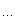Ikki's Story IV - Panda's Trick

Description

liympanda, one of Ikki’s friend, likes playing games with Ikki. Today after minesweeping with Ikki and winning so many times, he is tired of such easy games and wants to play another game with Ikki.

liympanda has a magic circle and he puts it on a plane, there are n points on its boundary in circular border: 0, 1, 2, …, n − 1. Evil panda claims that he is connecting m pairs of points. To connect two points, liympanda either places the link entirely inside the circle or entirely outside the circle. Now liympanda tells Ikki no two links touch inside/outside the circle, except on the boundary. He wants Ikki to figure out whether this is possible…

Despaired at the minesweeping game just played, Ikki is totally at a loss, so he decides to write a program to help him.

Input

The input contains exactly one test case.

In the test case there will be a line consisting of of two integers: n and m (n ≤ 1,000, m ≤ 500). The following m lines each contain two integers ai and bi, which denote the endpoints of the ith wire. Every point will have at most one link.

Output

Output a line, either “`panda is telling the truth...`” or “`the evil panda is lying again`”.

Sample Input

`4 20 13 2`

Sample Output

```panda is telling the truth...题意:给出n个构成一个环的点以及点跟点的连接关系。要求边不能相交。比如：有一条边i会跟j看成直线的话在园内相交，那么可以把一条通过边弯绕过圆，使得两条边不相交。最后问是否所有线段都不相交。分析：对于两条边，如果在园内相交，则必须引导一条通过园外。把边i看成点i1和i2分别表示通过园内和园外。如果i与j相交于园内，则i1->j2，i2->j1, j2->i1, j1->i2.表示两条边一条在园外，一条在园内。通过求scc确定每条边的i1和i2所在的scc是否相同，相同表示i既是在园内也是在园外，矛盾了。代码：
#include <stdio.h>#include <stdlib.h>#define Min(a, b) a < b ? a : b#define maxn 5010struct L{    int x, y;}line;struct g{    int v, next;}fn[maxn * 4];int g[maxn * 5], visit[maxn * 5], low[maxn * 5];void set(int m){    for (int i = 1; i <= m; i++)    {        g[i] = -1, visit[i] = 0;    }}int tarjan(int u, int f, int times){    low[u] = times;    visit[u] = 1;    int i, v;    for (i = g[u]; i != -1; i = fn[i].next)    {        v = fn[i].v;        if (v != f)        {            if (!visit[v])            {                times = tarjan(v, u, times + 1);            }            low[u] = Min(low[u], low[v]);        }    }    return times;}int main(){    int n, m, i, j, th, times;    scanf("%d%d", &n, &m);        set(m * 2);        for (i = 1; i <= m; i++)        {            scanf("%d%d", &line[i].x, &line[i].y);            if (line[i].x > line[i].y)            {                line[i].x ^= line[i].y, line[i].y ^= line[i].x, line[i].x ^= line[i].y;            }        }        for (i = 1, th = 0; i < m; i++)        {            for (j = i + 1; j <= m; j++)            {                //对相交的线段建图                 if (line[i].x < line[j].y && line[i].x > line[j].x && line[i].y > line[j].y                    || line[i].y < line[j].y && line[i].y > line[j].x && line[i].x < line[j].x)                {                    //printf("%d %d %d %d\n", line[i].x, line[i].y, line[j].x, line[j].y);                    fn[th].v = j + m, fn[th].next = g[i], g[i] = th++;                    fn[th].v = i, fn[th].next = g[j+m], g[j+m] = th++;                    fn[th].v = j, fn[th].next = g[i+m], g[i+m] = th++;                    fn[th].v = i + m, fn[th].next = g[j], g[j] = th++;                }            }        }//system("pause");        for (i = 1, times = 1; i <= 2 * m; i++)        {            if (!visit[i])            {                times = tarjan(i, -1, times + 1);            }            //printf("[%d]=%d ", i, low[i]);        }//printf("\n");        for (i = 1; i <= m; i++)        {            if (low[i] == low[i+m])            {                break;            }        }        if (i == m + 1)        {            printf("panda is telling the truth\n");        }        else        {            printf("the evil panda is lying again\n");        }    system("pause");    return 0;}/*10 31 52 67 3*/
```